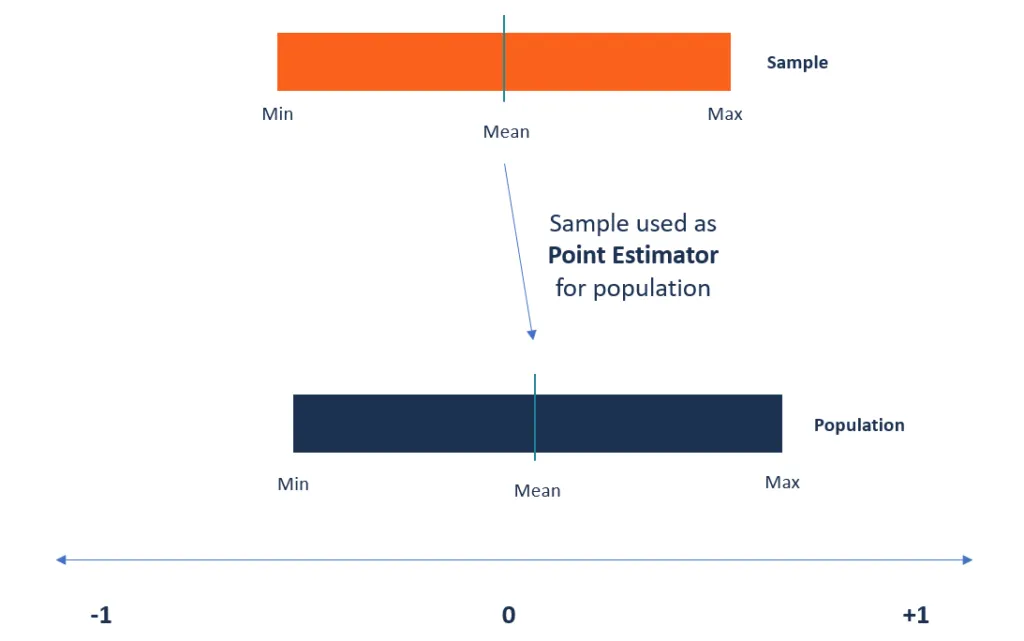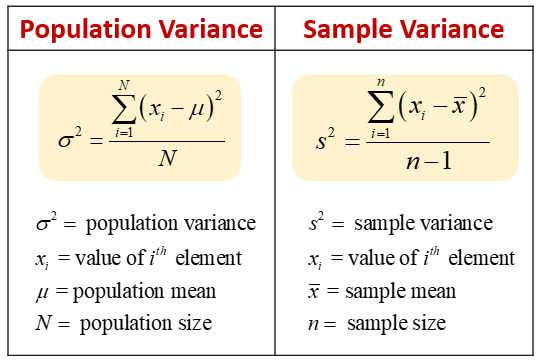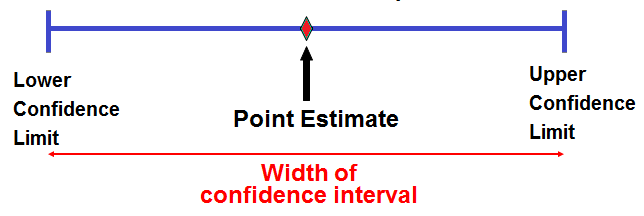Aashi Goyal — Published On May 31, 2021 and Last Modified On June 8th, 2021

## Introduction

Estimation Theory and Hypothesis testing are the very important concepts of Statistics that are heavily used by Statisticians, Machine Learning Engineers, and Data Scientists.

So, In this article, we will be discussing the Point Estimators in the Estimation Theory of Statistics.

1. Estimate and Estimators

2. What are Point Estimators?

3. What is Random Sample and Statistic?

4. Two common Statistic used:

• Sample mean
• Sample Variance

5. Properties of Point Estimators

• Unbiasedness
• Efficient
• Consistent
• Sufficient

6. Common methods of finding Point Estimates

7. Point Estimation vs Interval Estimation

## Estimate and Estimators

Let X be a random variable having distribution fx(x;θ), where θ is an unknown parameter. A random sample, X1, X2, ——, Xn, of size n taken on X.

The problem of point estimation is to pick a statistic, g(X1, X2, ——–, Xn), that best estimates the parameter θ.

Once observed, the numerical value of g(x1, x2, ——–, xn) is called an estimate, and Statistic g(X1, X2, ——–, Xn) is called an estimator.

## What is Point Estimators?

Point estimators are defined as the functions that are used to find an approximate value of a population parameter from random samples of the population. They take the help of the sample data of a population to determine a point estimate or a statistic that serves as the best estimate of an unknown parameter of a population.Most often, the existing methods of finding the parameters of large populations are unrealistic.

For example, when we want to find the average height of peoples attending a conference, it will be impossible to collect the exact height of every conference peoples in the world. Instead, a statistician can use the point estimator to estimate the population parameter.

## Random Sample and Statistic

Random Sample: A set of IID(Independent and Identically distributed) random variables, X1, X2, X3, ——–, Xn defined on the same sample space is called a random sample of size n.

Statistic: A function of a random sample is called a statistic (if it does not depend upon any unknown entity)

For Example, X1+X2+——+Xn, X12X2+eX3, X1– Xn

## Sample Mean and Sample Variance

Two important Statistic:

Let X1, X2, X3, ——–, Xn be a random sample, then:

The Sample mean is denoted by  x̄, and Sample Variance is denoted by s2

Here x̄ and s2 are called the sample parameters.

The population parameters are denoted by:

σ2 = population variance, and µ = population meanFig. Population and Sample MeanFig. Population and Sample Variance

Characteristics of sample mean:

E( x̄ ) = 1/n( Σ E(Xi) ) = 1/n (nµ) = µ

Var( x̄ ) = 1/n2( Σ Var(Xi) ) = 1/n2 (nσ2) = σ2/n

Characteristics of sample variance:

s2 = 1/(n-1) ( Σ ( xi– x̄ )2 ) = 1/(n-1) ( Σ xi2 – 2x̄ Σ xi + nx̄2 ) = 1/(n-1) ( Σ xi2 – nx̄2 )

Now, take the expectation on both sides, we get:

E(s2) = 1/(n-1) ( Σ E(xi2) – n E(x̄2) ) = 1/(n-1) ( Σ (µ22) – n(µ22/n)) = 1/(n-1) ((n-1)σ2) = σ2.

## Properties of Point estimators

In any given problem of estimation, we may have an infinite class of appropriate estimators to choose from. The problem is to find an estimator g(X1, X2, ——–, Xn), for an unknown parameter θ or its function Ψ(θ), that has “nice” properties.

Essentially, we would like the estimator g to be “close” to Ψ.

The following are the main properties of point estimators:

1. Unbiasedness:

Let’s first understand the meaning of the term “Bias”

The difference between the expected value of the estimator and the value of the parameter being estimated is referred to as the bias of a point estimator.

Therefore, the estimator is considered unbiased when the estimated value of the parameter and the value of the parameter being estimated is equal.

Also, the closer the expected value of a parameter is to the value of the parameter being measured, the lesser the value of the bias is.

Mathematically,

An estimator g(X1, X2, ——–, Xn) is said to be an unbiased estimator of θ if

E(g(X1, X2, ——–, Xn))= θ

That is, on average, we expect g to be close to the true parameter θ. We have seen that if X1, X2, ——–, Xn be a random sample from a population having mean µ and variance σ2, then

E( x̄ ) = µ and E(s2) = σ2

Thus, x̄ and s2 are unbiased estimators for µ and σ2

2. Efficient:

The most efficient point estimator is the one which is having the smallest variance of all the unbiased and consistent estimators. The variance represents the level of dispersion from the estimate, and the smallest variance should vary the least from one sample to the other.

Generally, the efficiency of the estimator depends on the distribution of the population.

Mathematically,

An estimator g1(X1, X2, ——–, Xn) is more efficient than g2(X1, X2, ——–, Xn), for θ if

Var(g1(X1, X2, ——–, Xn) ) <= Var(g2(X1, X2, ——–, Xn))

3. Consistent:

Consistency describes how close the point estimator stays to the value of the parameter as it increases in size. For it to be more consistent and accurate, the point estimator requires a large sample size.

We can also verify if a point estimator is consistent by looking at its corresponding expected value and variance.

For the point estimator to be consistent, the expected value should move toward the true value of the parameter.

Mathematically,

Let g1, g2, g3, ——- be a sequence of estimators, the sequence is said to be consistent if it converges to θ in probability i.e,

P( | gm(X1, X2, ——–, Xn)- θ| > ε ) -> 0 as m->∞

If X1, X2, ——–, Xn is a sequence of IID random variables such that E(Xi)= µ, then by WLLN (Weak Law of Large numbers):

Xn’—–>µ in probability

Where Xn’ is the mean of X1, X2, X3, ———, Xn

4. Sufficient:

Let be a sample from X~f(x;θ). If Y=g(X1, X2, ——–, Xn) is a statistic such that for any other statistic Z= h( X1, X2, ——–, Xn), the conditional distribution of Z, given Y=y does not depend upon θ, then Y is called a sufficient statistic for θ.

## Common methods of finding point estimates

The process of point estimation involves the utilization of the value of a statistic that is obtained with the help of sample data to determine the best estimate of the corresponding unknown parameter of the population. Several methods can be used to compute or determine the point estimators, and each technique comes with different properties. Some of the methods are as follows:

1. Method of Moments (MOM)

It starts by taking all the known facts about a population into considerations and then applying those facts to a sample of the population. Firstly, it derives equations that relate the population moments to the unknown parameters.

The next step is to draw a sample of the population to be used to estimate the population moments. The equations generated in step one are then solved with the help of the sample mean of the population moments. This gives the best estimate of the unknown population parameters.

Mathematically,

Consider a sample X1, X2, X3, ———, Xn from f(x;θ1, θ2, —–, θm) .The objective is to estimate the parameters θ1, θ2, —–, θm.

Let the population (theoretical) moments be α1, α2, ——–, αr, which are functions of unknown parameters θ1, θ2, —–, θm.

By equating the sample moments and population moments, we obtain the estimators of θ1, θ2, —–, θm.

2. Maximum Likelihood Estimator (MLE)

This method of finding point estimators tries to find the unknown parameters that maximize the likelihood function. It takes a known model and uses the values to compare data sets and find the most suitable match for the data.

Mathematically,

Consider a sample X1, X2, X3, ———, Xn from f(x;θ). The objective is to estimate the parameters θ (scalar or vector).

The likelihood function is defined as:

L(θ; x1, x2, ———, xn) = f(x1, x2, ———, xn;θ)

An MLE of θ is the value θ’ (a function of sample) that maximizes the likelihood function

If L is a differentiable function of θ, then the following likelihood equation is used to obtain the MLE (θ’):

d/dθ(ln(L(θ; x1, x2, ———, xn) =0

If θ is a vector, then partial derivatives are considered to get the likelihood equations.

## Point Estimation vs. Interval Estimation

Mainly, there are two main types of estimators in statistics:

• Point estimators
• Interval estimators

Point estimation is the opposite of interval estimation.

Point Estimation generates a single value while Interval Estimation generates a range of values.

A point estimator is a statistic that is used to estimate the value of an unknown parameter of a population. It uses sample data from the population when calculating a single statistic that will be considered as the best estimate for the unknown parameter of the population.On the contrary, interval estimation takes sample data to determine the interval of the possible values of an unknown parameter of a population. The interval of the parameter is selected in a way that it falls within a 95% or higher probability, also known as the confidence interval. The confidence interval describes how reliable an estimate is, and it is calculated from the observed data. The endpoints of the intervals are known as the upper and lower confidence limits.

## Endnotes

I hope you enjoyed the article and increased your knowledge about the Estimation Theory.

Please feel free to contact me on Email

Something not mentioned or want to share your thoughts? Feel free to comment below And I’ll get back to you.